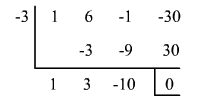# The Factor Theorem

Generally when a polynomial is divided by a binomial there is a remainder.

Consider the polynomial function $f\left(x\right)={x}^{3}+6{x}^{2}-x-30$ . Divide the polynomial $f\left(x\right)$ by the binomial $x+3$ .

###Observe that, the remainder is $0$ .

When you divide a polynomial by one of its binomial factors, the quotient is called a depressed polynomial.

Here the quotient or the depressed polynomial is ${x}^{2}+3x-10$ .

From the results of the division and by using the Remainder Theorem , we can write the following statement.

${x}^{3}+6{x}^{2}-x-30=\left({x}^{2}+3x-10\right)\left(x+3\right)+0$ .

Since the remainder is $0$ , the function value at $-3$ is $0$ or $f\left(-3\right)=0$ . This means that the binomial $x+3$ is a factor of the polynomial function $f\left(x\right)={x}^{3}+6{x}^{2}-x-30$ .

This illustrates the Factor Theorem.

A polynomial $f\left(x\right)$ has a factor $\left(x-k\right)$ if and only if $f\left(k\right)=0$ where $f\left(x\right)$ is a polynomial of degree $n\ge 1$ and $k$ is any real number.